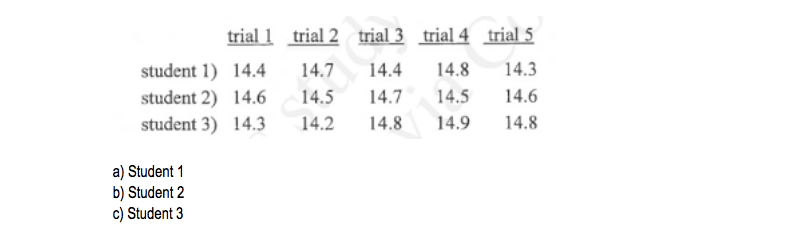# Problem: Consider the data obtained for the length of an object as measured by three students. The length is known to be 14.49350 cm. Which student has done the most accurate determination?

###### FREE Expert Solution
86% (443 ratings)
###### Problem Details

Consider the data obtained for the length of an object as measured by three students. The length is known to be 14.49350 cm. Which student has done the most accurate determination?What scientific concept do you need to know in order to solve this problem?

Our tutors have indicated that to solve this problem you will need to apply the Accuracy & Precision concept. You can view video lessons to learn Accuracy & Precision. Or if you need more Accuracy & Precision practice, you can also practice Accuracy & Precision practice problems.

What is the difficulty of this problem?

Our tutors rated the difficulty ofConsider the data obtained for the length of an object as me...as medium difficulty.

How long does this problem take to solve?

Our expert Chemistry tutor, Jules took 1 minute and 41 seconds to solve this problem. You can follow their steps in the video explanation above.

What professor is this problem relevant for?

Based on our data, we think this problem is relevant for Professor Davidson's class at UTSA.